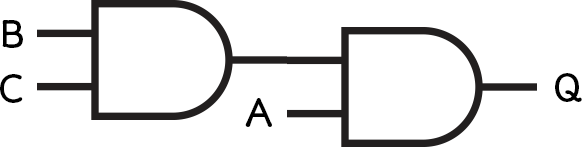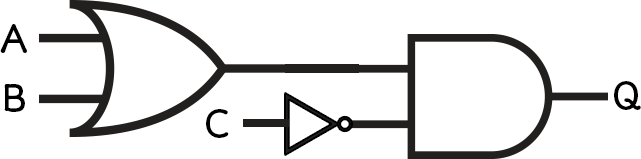### Computational Thinking, Algorithms & Programming

Boolean Logic
Computational Thinking
Algorithm Design
Sequence, Selection & Iteration
Data Structures
Sub Programs
Producing Robust Programs
Programming Languages & IDEs
Searching & Sorting Algorithms

# Combining Gates & Solving Problems

We have seen how logic circuits can be drawn as diagrams with inputs and outputs, and we can use truth tables to map out the range of possible results from these circuits.

However, we can also combine a series of logic gates to produce more complex circuits. This allows us to solve problems and identifies logical structures in how a computer works.

In this lesson, we’ll learn about:

1. Combining logic gates to produce simple circuits.
2. Applying logical diagrams & operators in truth tables to solve problems.
Media Attachments:

## 1. Producing Simple Circuits

We can start to put these logic gates together to make larger circuits. Each circuit can be represented as a Boolean expression, which will always start with an output, usually called Q.

To solve these, start with the brackets on the inside and work outwards.

### Worked Example 1

Q = A AND (B AND C)

Step 1 – Start with the brackets, this is the “B AND C” part.

Step 2 – Add the outer expression, this is the “A AND” part.Figure 2 – the result of B AND C is used as input on another AND gate with A.

### Worked Example 2

Q = NOT (A OR B)

Step 1 – Start with the brackets, this is the “A OR B” part.

Step 2 – Add the outer expression, this is the “NOT” part.

### Worked Example 3

Q = (A OR B) AND NOT C

Step 1 – Start with the brackets, this is the “A OR B” part.

Step 2 – Add the outer expression, this is the “AND NOT C” part.Figure 6 – the result of A OR B is used as input on an AND gate with NOT C.

### Further Thought

Can you double NOT a circuit? Research this online.

## 2. Solving Problems

We have seen how we can use Boolean algebra to represent some circuits. We can build up truth tables to show all the inputs and all the possible outputs for any circuit. We can then use this to work out which combination of inputs will give us a TRUE output.

The way to do this is to start small and build up your truth table column by column.

In your exam you might need to complete a truth table for a given Boolean expression, or draw the circuit diagram for a Boolean expression and then plot the truth table.

In order to solve this you can follow some very simple steps:

2. Check to see if any of your inputs have been NOTed
4. Look at the final output

### Worked Example 1

Let’s look at a simple Boolean expression, Q = A AND NOT B.

#### Step 1 – Build your inputs

We have two inputs A and NOT B. So we need 4 rows.

#### Step 2 – Check to see if any of your inputs have been NOTed

Yes – B has been NOTed, so add a column for NOT B.

Notice how all we have done is flip the B column values.

Now we need to add the expression by comparing the A column and the NOT B column.

As it is an AND gate, the output will be true if both inputs are true.

#### Step 4 – Look at the final output

Our expression is Q = A AND NOT B, so the values in column four is our answer.

What this means is that there is only one possible input to this circuit that gives a TRUE output. When A = 1 and B = 0.

### Worked Example 2

Consider the following expression, Q = (A OR B) AND C.

#### Step 1 – Build your inputs

First, we have three inputs, so let’s see the truth table outline. We should have 8 rows this time.

#### Step 2 – Check to see if any of your inputs have been NOTed

They haven’t so we can skip this step.

First, we have A OR B – ignore C for the moment.

Now we can add the AND C part.

Remember it’s AND – so it’s where we have a 1 in the A OR B column AND we have a 1 in the C column.

#### Step 4 – Look at the final output

Our expression is Q = (A OR B) AND C, so the values in column five is our answer.

This is a quite complex circuit. There are THREE combinations of A, B and C that will give us a TRUE output (remember this is like the lights going on).

The following table shows the combinations of A, B and C that give us a TRUE output.

### Worked Example 3

Consider the following expression, Q = (A AND NOT B) OR (A AND NOT C).

#### Step 1 – Build your inputs

First, we have 3 inputs so let’s see the truth table outline. We should have 8 rows.

#### Step 2 – Check to see if any of your inputs have been NOTed

Yes – we have NOT B and NOT C, so we add these columns.

First, we have A AND NOT B, so we add this column – again the output is TRUE when both inputs are TRUE, as it is an AND gate.

Now let’s add A AND NOT C.

Now we can build up our final expression where we have a 1 in the (A AND NOT B) column OR we have a 1 in the (A AND NOT C) column.

### Step 4 – Look at the final output

Our expression is Q = (A AND NOT B) OR (A AND NOT C), so the values in column eight is our answer.

So here we can see that there are only 3 possible inputs to this gate that give us an output of true:

### Further Thought

Why would programmers use truth tables to work out complex logical IF ELSE statements?

## Lesson Summary

So to summarise what we’ve learnt in this lesson:

• You can combine logic gates to draw Boolean expressions.
• Truth tables can be used to work out the outputs of any Boolean circuit.
• They can be used to work out the Boolean algebra of any circuit.
• The truth tables follow four rules.
• Step 1 – Build your inputs
• Step 2 – Check to see if any of your inputs have been NOTed